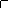WOW !! MUCH LOVE ! SO WORLD PEACE !
Fond bitcoin pour l'amélioration du site: 1memzGeKS7CB3ECNkzSn2qHwxU6NZoJ8o
Dogecoin (tips/pourboires): DCLoo9Dd4qECqpMLurdgGnaoqbftj16NvpRechercher sur le site:

# Equations differentials stochastics involving fractional brownian motion two parameter

( Télécharger le fichier original )
Université de Saà¯da - Master 2012

University of Saïda
Faculty of Sciences and Technology
Department of Mathematics & Computer machine
Probability & Applications

Memory of Master

Equations Differentials Stochastics

Involving Fractional Brownian Motion

Two-parameter

Si tu veux courir, cours un kilomètre, si tu veux
changer ta vie, cours un marathon
Emil Zatopek1.

# Contents

1 Element of Fractional Brownian Motion 5

1.1 Fractional Brownian Motion 5

1.1.1 Self-similarity 5

1.1.2 Hölder Continuity 6

1.1.3 Path Differentiability 7

1.1.4 The Fractional Brownian Motion is not a SemimartingaleforH=61 2 7
1.1.5 Fractional Integrals and Fractional Derivatives of Func-

tions 8

1.2 Two-parameter Fractional Brownian Motion 9

1.2.1 The Main Definition 9

1.2.2 Fractional Integrals and Fractional Derivatives of Two-

parameter Functions 10

1.2.3 Hölder Properties of Two-parameter fbm 12

2 Stochastic Integration with Respect to Two-parameter Fractional Brownian Motion 15

2.1 Pathwise Integration in Two-parameter Besov Spaces 15

2.2 Some Additional Properties 23

3 Existence and Uniqueness of the Solutions of SDE with Two-Parameter Fractional Brownian Motion 25

4 CONTENTS

# Motion

## 1.1 Fractional Brownian Motion

Definition 1.1.0.1. The (two-sided, normalized) fractional Brownian motion (fBm) with Hurst index H E (0, 1) is a Gaussian process BH = {BH t , t E R} on (Ù, F, P), having the properties:

1. BH 0 = 0,

2. EBH t = 0; t E R,

1 (|t|2H + |s|2H - |t - s|2H) ; t, s E R,

3. EBH t BH s = 2

### 1.1.1 Self-similarity

Definition 1.1.1.1. We say that an Rd-valued random process X = (Xt)t=0 is self-similar or satisfies the property of self-similarity if for every a > 0 there exist b > 0 such that:

law (Xat, t = 0) = law (bXt, t = 0) (1.1)

Note that (1.1) means that two process Xat and bXt have the same finite-dimensional distribution functions, i.e., for every choice t1, ..., tn E R,

P (Xat0 = x0, ..., Xatn = xn) = P(bXt0 = x0, ..., bXtn = xn) For every x0, ..., xn E R.

Definition 1.1.1. A stochastic process X = {Xt, t E R} is called b-selfsimilar if

{Xat,t E R} d ={abXt,t E R} in the sense of finite-dimensional distributions.

### 1.1.2 Hölder Continuity

We recall that according to the Kolmogorov criterion , a process X = (Xt)t?R admits a continuous modification if there exist constants á = 1, â > 0 and k > 0 such that

E [|X(t) - X(s)|á] = k|t - s|1+â

for all s,t E R.

Theorem 1.1.2.1. Let H E (0, 1). The fractional brownian motion BH admits a version whose sample paths are almost surely Hölder continuous of order strictly less than H.

Proof. We recall that a function f : R -? R is Hölder continuous of order á, 0 < á = 1 and write f E Cá(R), if there exists M > 0 such that

|f(t) - f(s)| = M|t - s|á,

For every s, t E R. For any á > 0 we have

E ~|BH t - BH t |á] = E [|BH 1 |á] |t - s|áH;

Hence, by the Kolmogorov criterion we get that the sample paths of BH are almost everywhere Hölder continuous of order strictly less than H. Moreover, by  we have

 lim t-+0+ sup ~~BH ~ (t) = CH tHs/log log t-1with probability one, where CH is a suitable constant. Hence BH can not have sample paths with Hölder continuity's order greater than H.